# Seating Arrangements Notes | Study Business Mathematics and Logical Reasoning & Statistics - CA Foundation

## CA Foundation: Seating Arrangements Notes | Study Business Mathematics and Logical Reasoning & Statistics - CA Foundation

The document Seating Arrangements Notes | Study Business Mathematics and Logical Reasoning & Statistics - CA Foundation is a part of the CA Foundation Course Business Mathematics and Logical Reasoning & Statistics.
All you need of CA Foundation at this link: CA Foundation

BASED ON VARIOUS PATTERN OF SITTING ARRANGEMENTS

ARE CLASSIFIED INTO
1) Linear Arrangements
2) Circular Arrangements
3) Polygon Arrangements
Here we are limited to our topic linear and circular arrangements only. While making arrangements, it should be noted that all the conditions given are compiled with. These type of questions generally involve five to eight individuals arranged in a certain manner or pre-conditions. They may have to be arranged in a Circle or in a row accordingly.

Sometimes these questions are made more difficult by allowing an individual to a particular position with some conditions.
General instructions to Solve Seating Arrangement Questions are as follows.
1) First of all take a review on the given information. After performing this step, you would get an idea of the situation of people or objects.
2) Next, determine the usefulness of each information’s and classify them accordingly into ‘definite information’, ‘comparative information’ and ‘negative information’.
3) When the place of any objects or persons is definitely mentioned then we say that it is a definite information, X is sitting on the right end of the bench.
4) When the place of any object or person is not mentioned definitely but mentioned only in the comparison of another person or object, then we say that it is a comparative information.
Example 1: A is sitting second to the right of E. This type of information can be helpful when we can get the definite information about E.
5) A part of definite information may consist of negative information. A negative information does not tell us anything definitely but it gives an idea to eliminate a possibility.
Example 2: C is not sitting on the immediate left of A.

TYPE-1 LINEAR ARRANGEMENT
In this type of arrangement, we arrange objects or persons in a line or row. The arrangement is done only on one ‘axis’ and hence, the position of persons or objects assumes importance in terms of order like positions. In this type of arrangement, we take directions according to our left and right.
Steps to Solve the Linear Arrangements:
(a) Identify the number of objects and their names.
(b) Use pictorial method to represent the people or objects and their positions.
(c) Arrange the information with relevant facts and their positions and try to find out the solution.
There are few words which must be paid adequate attention, i.e., ‘between’ means sandwiched, ‘immediate left’ is different from ‘to the left’. To understand it let us see some pictorial representation.
When direction of face is not clear, then we take One Row Sequence
(A) When direction of face is not clear, then we take based on diagram will be as follows: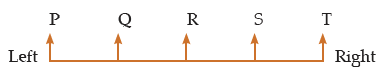From the above diagram, it is clear that
(i) Q, R, S, T are right of P but only Q is the immediate right of P.
(ii) S, R, Q, P are left of T but only S is the immediate left of T.
(iii) R, S, T are right of Q only R is the immediate right of Q.
(iv) R, Q, P are left of S but only R is the immediate left of S.
(v) S and T are right of R but only S is the immediate right of R.
(vi) Q and P are left of R but only Q is the immediate left of R.
(vii) A is the immediate left of Q while T is the immediate right of S.
(B) When direction of face is towards you, then the diagram will be as follows: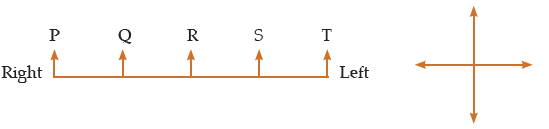From the above diagram, it is clear that
(i) Left of P = P, R, S and T
(ii) Right of T = S, R, Q and P
(iii) Q is immediate left of P; R is immediate left of Q; S is immediate left of R and T is immediate left of S.
(iv) S is immediate right of T; R is immediate right of S; Q is immediate right of R; and P is immediate right of Q.
Two Rows Sequence
Let us see 6 persons seating in two rows.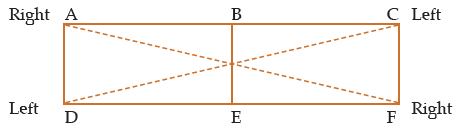From the above diagram, it is clear that
(i) A is sitting opposite D
(ii) B is sitting opposite E
(iii) C is sitting opposite F
(iv) D and C are sitting at diagonally opposite positions
(iv) S and R are sitting at diagonally opposite positions.
Example 3: Four Children’s are sitting in arrow. A is occupying seat next to B but not next to C. If C is not sitting next to D? Who is occupying seat next to adjacent to D.
(a) B
(b) B and A
(c) Impossible to tell
(d) A
Solution: (d) The arrangements as per given information is possible only if C is sitting next to B and D is sitting next to A.
Therefore, two possible arrangements are C, B, A, D, or D, A, B, C
Clearly, only A is sitting adjacent to D:
Example 4: P, Q, R, S, T, U, V and W are sitting in a row facing North.
(i) P is fourth to the right of T
(ii) W is fourth to the left of S
(iii) R and U, which are not at the ends, are neighbours of Q and T respectively.
(iv) W is next to the left of P and P is the neighbour of Q, who are sitting at the extreme ends
Solution:
From information
(i) we get that there are three persons between P and TXXXP.
In the information (iv), it is given that W is next to the left of P and Q is the neighbour of P. Using the information with (i), we get TXXWPQ.
By the information (ii), TXXWPQXS
By the information (iii),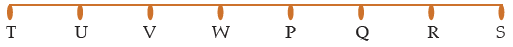So, T and S are sitting at the extreme ends.
Example 5: There are Five houses P, Q , R, S, T . P is right of Q and T is left of R and right  of P . Q is right of S. Which house in the middle.
(a) P
(b) Q
(c) R
(d) T

Solution: According to the question the houses can be arranged as follows.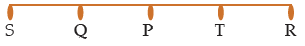Therefore, house P is middle.
Example 6: Friends are sitting on a bench. A is to the left of B but on the right of C, D is to the right of B but one the left of E. Who are at the extremes?

(a) A, B
(b) A, D
(c) C, E(d) B, D
Solution: Arrangements according to the question as follows.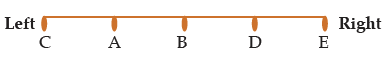Clearly C and E are the extremes.
Example 7: In a college party, 5 girls are sitting in a row. F is to the left of M and to the right of O. R is sitting to the right of N but to the left of O. Who is sitting in the middle?
(a) O
(b) R
(c) P
(d) M
Solution: (a) arrangements of the question as follows.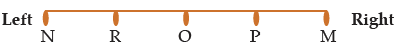Therefore, O is sitting in the middle.
Example 8: Five boys A, B, C, D and E are standing in a row. D is on the right of E, B is on the left of E but on the right of A. D is one the left of C, who is standing on the extreme right. Who is standing in the middle?
(a) B
(b) C
(c) D
(d) E
Solution: The sequence of Boys as follows.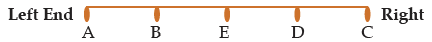There E is standing in the middle.

Circular Arrangement:

In this arrangement, some persons are sitting around a circle and they are facing the centre.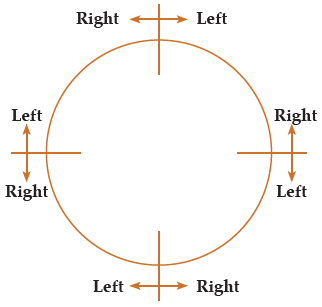1. Left movement is called clockwise rotation.
2. Right movement is called anti–clockwise rotation.
(i) The above presentation is for 4 persons but for any number of persons, the direction is taken in the same manner.
(ii) For rectangular and sequence arrangement, directions are taken as discussed in two rows sequence.
Example 9: (Q Nos. 1 to 3) Study the following Question carefully and answer the given questions. Four ladies & A, B, C and D and Four Gentlemen E, F, G and H are sitting in a circle around a table facing each other .
I. No two ladies or gentlemen are sitting side by side.
II. C, who I sitting between G and E, is facing D.
III. F is between D and A and facing G.
IV. H is to the right of B.
(1) Who is sitting left of A?
(a) E
(b) F
(c) G
(d) H
(2) E is facing whom?

(a) F
(b) B
(c) G
(d) H

(3) Who is immediate neighbour of B?
(a) G and H
(b) E and F
(c) E and H
(d) F and H
Solution: On the basis of given information in the question, the seating arrangements of the persons are as follows.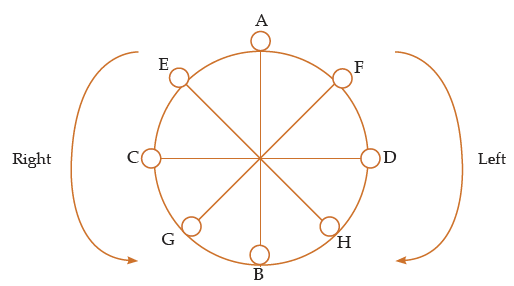1) (b) Clearly , F is sitting left of A.
2) (d) Clearly E is facing H.
3) (a) G and H are neighbours of B.
Example 10: Eight persons A, B, C, D, E, F, G and H are sitting around the circle as given in the figure. They are facing the direction opposite to centre. If they move upto three places anti-clockwise, then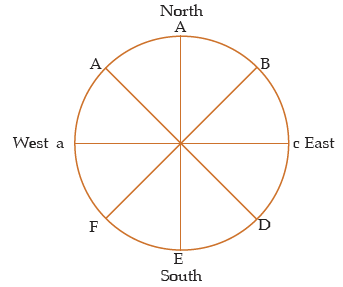(a) B will face west
(b) E will face East
(c) H will face North-West
(d) A will face South
Solution: Following Seating arrangement is formed from the given in formation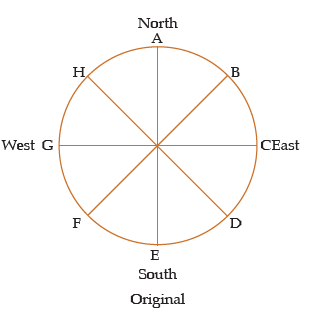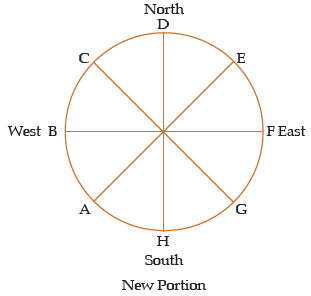Clearly B will Face west
Example 11: Five People A, B, C, D and E are seated about a round table. Every chair is spaced equidistant from adjacent chairs. (UPSC (CSAT) 2013)
I. C is seated next to A .
II. A is seated two seats from D.
III. B is not seated next to A.
Which of the following must be true?
I. D is seated next to B.
II. E is seated next to A.
Select the correct from the options given below:
(a) Only I
(b) Only II
(c) Both I and II
(d) Neither I nor II
Solution:
According to the given information there are possible Seating arrangements.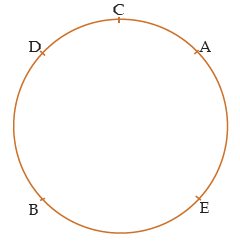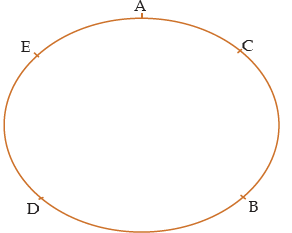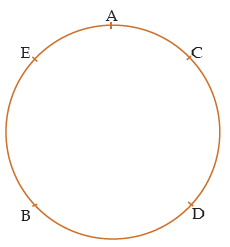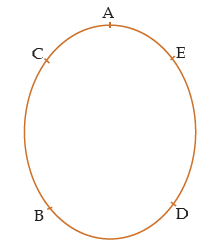From the above arrangements. It is clear that D is seated next to B . Also E is next to A.

Clearly both statements I and II are true.
Example 12: Study the following Question carefully and answer the given questions.
Eight friends A, B, C, D,E, G and H are sitting in a circle facing the centre, not necessarily in the same order. D sits third to the left of A. E sits to the immediate right of A. B is third to left of D. G is second to the right of B. C is neighbour of B. C is third to left of H. (GIC 2012)
1) Who amongst the following is sitting exactly between F and D?
(a) C
(b) E
(c) H
(d) A
2) Three of the following four are alike in a certain way based on the information given above and so form a group. Which is does not belong to that group.
(a) DC
(b) AH
(c) EF
(d) DF
3) Who amongst the following second to the left of H?
(a) E
(b) B
(c) A
(d) Noe of these
4) Who amongst the following are immediate neighbours of G?
(a) CA
(b) AF
(c) DC
(d) DF
5) Who amongst the following is sitting third to the right of A?
(a) F
(b) B
(c) H
(d) C
Solution: Arrangements according to the question is as follows.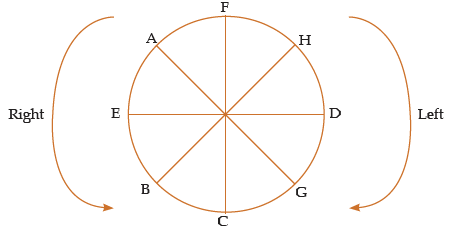1) (c), Clearly H is sitting exactly F and D
2) (d)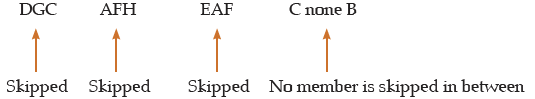So, CB does not belong to the group.

3) (d) Clearly, H is sitting exactly between F and D

4) (c) Clearly D and C immediate neighbours of G

5) (d) Clearly, C is sitting third to the right of A

The document Seating Arrangements Notes | Study Business Mathematics and Logical Reasoning & Statistics - CA Foundation is a part of the CA Foundation Course Business Mathematics and Logical Reasoning & Statistics.
All you need of CA Foundation at this link: CA FoundationUse Code STAYHOME200 and get INR 200 additional OFF

## Business Mathematics and Logical Reasoning & Statistics

81 videos|101 docs|87 tests

Track your progress, build streaks, highlight & save important lessons and more!

,

,

,

,

,

,

,

,

,

,

,

,

,

,

,

,

,

,

,

,

,

;